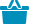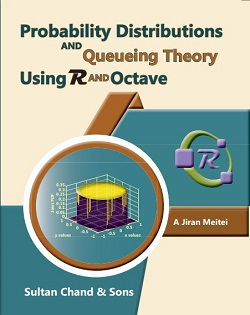Cart

# Probability Distributions and Queueing Theory Using R and Octave#### Quick Overview

Authored By

Publisher: Sultan Chand & Sons

Publishing Year: 2023

Size (mm): 240.00 x 185.00

ISBN: 93-91820-17-6

Page nos.:

MRP: 550.00

Subject: Mathematical Sciences

₹550.00

This book enables learners of Statistics, Mathematics, Operational Research, Economics, Commerce, and related disciplines to understand univariate probability distributions using R and Octave. The explanations and presentations in the book are simple and user-friendly. The book also focuses on Stochastic Process and Queueing Theory. Ample examples and exercise questions supplement the discussions in each chapter. All the statistical tables in the book are generated using the R (R codes are included for readers). The extensive use of MS Excel for working with distributions is another added feature.

Salient Features

• The book covers all the major univariate probability distributions and the basics of stochastic processes along with the Markovian queueing systems.
• The New Educational Policy framework promotes the use of open-source softwares. R and Octave are two powerful open-source softwares which are freely accessible on the internet.
• Each distribution is explained using R, Octave and MS Excel.
• All chapters are supplemented with ample examples.
• The book’s content will be helpful to students of statistics, mathematics, operational research, business economics, and management.

• Random Variables
• Mathematical Expectation
• Special Univariate Discrete Probability Distributions
• Special Univariate Continuous Probability Distributions-I
• Special Univariate Continuous Probability Distributions-II
• Sampling Distributions
• Fitting of Distributions
• Stochastic Processes and Basics of Queueing Theory
• Markovian Queues

ISBN13: 978-93-91820-17-6

Weight: 0.00

Edition: 1st Edition

Language: English

Title Code: 1284

#### Author

Authored By : Meitei Jiran A (Dr)